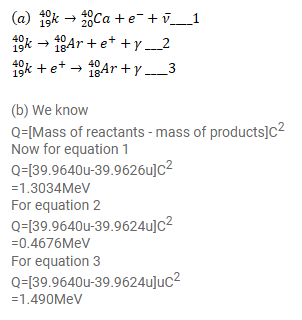# Potassium -40 can decay in three modes.

Question:

Potassium $-40$ can decay in three modes. It can decay by $\beta$-emission, $\beta^{+}$-emission or electron capture.

(a) Write the equations showing the end products.

(b) Find the Q-values in each of the three cases. Atomic masses of $1840 \mathrm{Ar}, 1940 \mathrm{~K}$ and $2040 \mathrm{Ca}$ are $39.9624 \mathrm{u}, 39.9640 \mathrm{u}$ and $39.9626 \mathrm{u}$ respectively.

Solution: